## General Question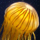# Can anyone help me with this? Chlorine and carbon monoxide gases are mixed in a 1.00L container and the following equilibrium is established: CO(g) + Cl2(g) <--> COCl2(g).. Initially 1.5 mol of chlorine was present with excess carbon monoxide. At equilibrium, 0.8 mol of COCl2(g) was found. How do you calculate the percent reaction?

Asked by cdog1122 (1) April 19th, 2009
Observing members: 0Composing members: 0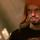For a start, your equation isn’t balanced; it should be Cl2 (Cl2, not 2Cl, because of HONClBrIF). Given this, if we assume the reaction goes to completion, 1.5 mol Cl2 will produce 1.5 mol COCl2. Now you just want the percent that was produced compared to the theoretical maximum that I just stated. So, .8/1.5*100=53.3%
Incidentally, is that supposed to be CO or Co? It has no effect on the calculation, just curious.

Jayne (6771)“Great Answer” (5) Flag as…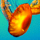Do you mean calculate how much of the Cl was consumed? Cuz that seems pretty simple….. Offhand looking at it quickly it looks like you need to make an ICE chart for this question.

FrankHebusSmith (4319)“Great Answer” (1) Flag as…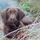Well, I guess Jayne & westy told you…...........X2!

Cardinal (2926)“Great Answer” (0) Flag as…@westy81585; I think the equilibrium and ICE chart are irrelevant, because he gives us no K value for the reaction. So unless we are expected to look it up, I assume that the question is meant to be a basic stoichiometry problem.

Jayne (6771)“Great Answer” (0) Flag as…do i assume that because of the molar ratio, that there is also 1.5 mol of CO(g) needed for reaction?

cdog1122 (1)“Great Answer” (0) Flag as…Yes; however much is consumed of the one, the same must be consumed of the other. Also, the same amount of product must be formed (assuming they all react on a 1-to-1 ratio; that is, if the coefficients are the same. If not, then the amount used by a substance with a coefficient of 1 is multiplied by the coefficient of each substance to find the amount used/produced of that substance. See second paragraph). This is true no matter if the reaction proceeds to equilibrium or if it stops at a midway equilibrium (everything does, of course, but some go so close to completion as to make no difference). In this case, of course, because we are dealing with an equilibrium, and the reaction does not go to completion; since .8 mol product were formed, .8 mol of Cl2 was used, and .8 mol CO as well.

And if the formula were somehow 2CO+Cl2, then two moles of CO would be needed for every mole of Cl2. So, 3 moles would be used; or rather, 1.6, because of equilibrium.

Because of this, if in the first case there was 2 mol CO and 3 mol Cl2, then at the end there would be 2 mol COCl2 and 0 mol CO, and there would remain 1 mol Cl2. That 1 Cl2 has nothing to react with, and so it hangs around (according to the formula, at least). Thus, CO “limits” the reaction; it is called the limiting reagent. In the second case I gave, even if there were 3 mol CO and 2 mol Cl2, CO is still the limiting reagent, because it takes 2 moles of CO to react with 1 mole of Cl2, so if 2 mol Cl2 can form 2 mol product, 3 mol CO can only form 1.5. So to find the limiting reagent, just calculate the amount of product that each reactant can possibly form, given their amounts, and take the lowest one.

Jayne (6771)“Great Answer” (0) Flag as…augustlan (47720)“Great Answer” (0) Flag as…Yah I agree with Jayne on this. My ICE idea would be pointless without a rxn rate (k).

@ augustian Come on, it’s just basic chem :P

FrankHebusSmith (4319)“Great Answer” (0) Flag as…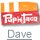I was going to say Fluther doesn’t do your homework for you, but looks like I’m wrong.

PupnTaco (13885)“Great Answer” (0) Flag as…Unfortunately, that is more telling of my ability to explain than of your ability to do chemistry. I’m sure this would all seem basic to you if I could state it properly.

@Dave; I’m not going to do a whole assignment for him. But asking for help on one problem is a perfectly legitimate way to learn how to do something, and I’m happy to pass on what little knowledge I have on a subject.

Incidentally, the first “equilibrium” in the third sentence should be “reaction”, and the last paragraph is meant as a side note- I would have put it in whisper, but then it would have been even more eye-watering.

Jayne (6771)“Great Answer” (0) Flag as…or